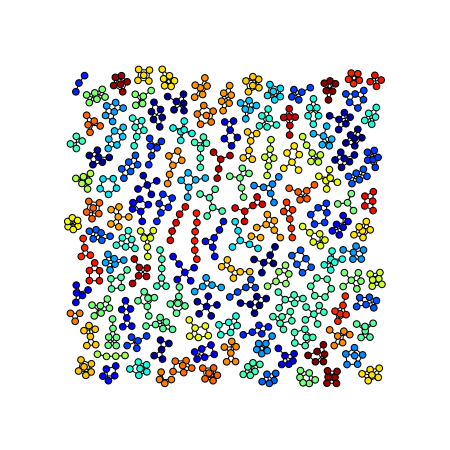Warning

This documents an unmaintained version of NetworkX. Please upgrade to a maintained version and see the current NetworkX documentation.

# Atlas¶#!/usr/bin/env python
"""
Atlas of all graphs of 6 nodes or less.

"""
__author__ = """Aric Hagberg (hagberg@lanl.gov)"""
#    Copyright (C) 2004 by
#    Aric Hagberg <hagberg@lanl.gov>
#    Dan Schult <dschult@colgate.edu>
#    Pieter Swart <swart@lanl.gov>

import networkx as nx
from networkx.generators.atlas import *
from networkx.algorithms.isomorphism.isomorph import graph_could_be_isomorphic as isomorphic
import random

def atlas6():
""" Return the atlas of all connected graphs of 6 nodes or less.
Attempt to check for isomorphisms and remove.
"""

Atlas=graph_atlas_g()[0:208] # 208
# remove isolated nodes, only connected graphs are left
U=nx.Graph() # graph for union of all graphs in atlas
for G in Atlas:
zerodegree=[n for n in G if G.degree(n)==0]
for n in zerodegree:
G.remove_node(n)
U=nx.disjoint_union(U,G)

# list of graphs of all connected components
C=nx.connected_component_subgraphs(U)

UU=nx.Graph()
# do quick isomorphic-like check, not a true isomorphism checker
nlist=[] # list of nonisomorphic graphs
for G in C:
# check against all nonisomorphic graphs so far
if not iso(G,nlist):
nlist.append(G)
UU=nx.disjoint_union(UU,G) # union the nonisomorphic graphs
return UU

def iso(G1, glist):
"""Quick and dirty nonisomorphism checker used to check isomorphisms."""
for G2 in glist:
if isomorphic(G1,G2):
return True
return False

if __name__ == '__main__':

import networkx as nx

G=atlas6()

print("graph has %d nodes with %d edges"\
%(nx.number_of_nodes(G),nx.number_of_edges(G)))
print(nx.number_connected_components(G),"connected components")

try:
from networkx import graphviz_layout
except ImportError:
raise ImportError("This example needs Graphviz and either PyGraphviz or Pydot")

import matplotlib.pyplot as plt
plt.figure(1,figsize=(8,8))
# layout graphs with positions using graphviz neato
pos=nx.graphviz_layout(G,prog="neato")
# color nodes the same in each connected subgraph
C=nx.connected_component_subgraphs(G)
for g in C:
c=[random.random()]*nx.number_of_nodes(g) # random color...
nx.draw(g,
pos,
node_size=40,
node_color=c,
vmin=0.0,
vmax=1.0,
with_labels=False
)
plt.savefig("atlas.png",dpi=75)